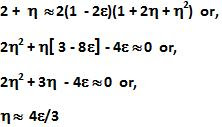## Wednesday, January 6, 2010

### Irodov Problem 1.374

Let T be the kinetic energy of the particle. Let its rest mass be mo. Let the ratio of its kinetic energy to rest mass energy be equal to,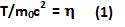If we used Newtonian mechanics to determine the velocity we would have,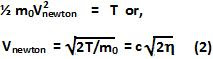If we used Relativistic mechanics on the other hand we would have,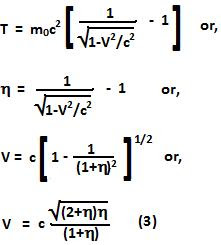The relative error in the estimation of velocity would then be,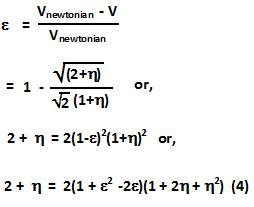For small values ofwe can consider only the linear terms in equation (4) and we have,